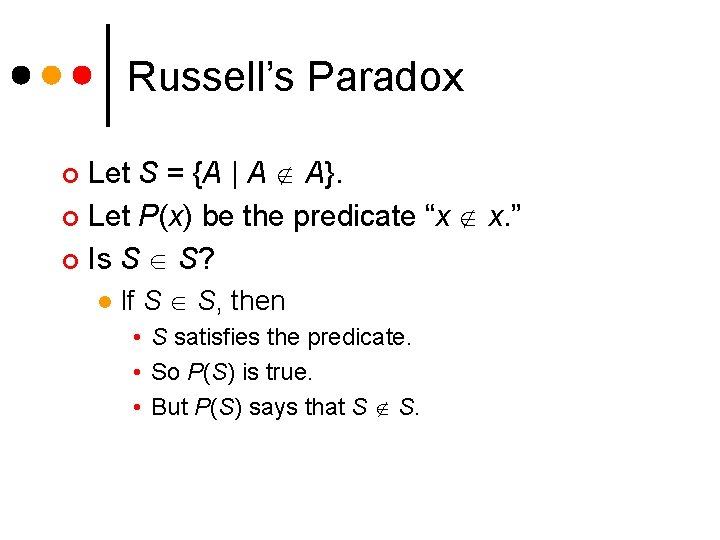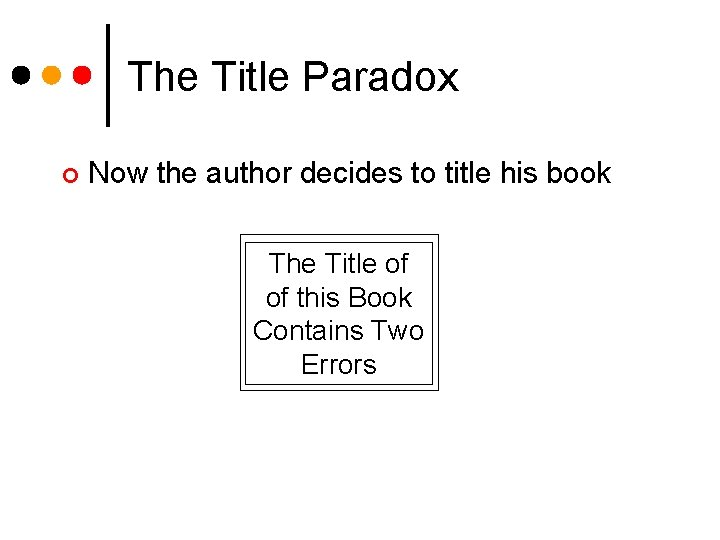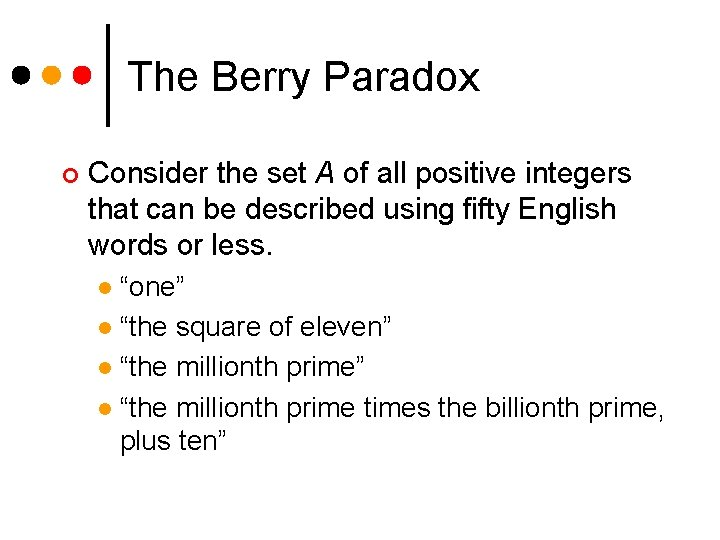# Russells Paradox Lecture 24 Section 5 4 Fri

• Slides: 11
Download presentationRussell’s Paradox Lecture 24 Section 5. 4 Fri, Mar 10, 2006Russell’s Paradox Let A be any set that you have ever seen. ¢ Then, most likely, A A. ¢ For example ¢ A set of integers is not itself an integer. l A set of rectangles is not itself a rectangle. l A set of points in the plane is not itself a point in the plane. l ¢ Is it possible that A A for some set A?Russell’s Paradox Let S = {A | A A}. ¢ Let P(x) be the predicate “x x. ” ¢ Is S S? ¢ l If S S, then • S satisfies the predicate. • So P(S) is true. • But P(S) says that S S.Russell’s Paradox l If S S, then • S does not satisfy the predicate. • So P(S) is false • But that means that S S. Therefore, “S S” is neither true nor false. ¢ This is a paradox. ¢The Barber Paradox In a certain town, there is a barber who cuts the hair of every person (them and only them) in the town who does not cut his own hair. ¢ Question: Who cuts the barber’s hair? ¢The Bibliography Paradox An author writes a book about bibliographies. ¢ He decides to list in the bibliography of this book all books that do not list themselves in their own bibliographies. ¢ Should he list his own book? ¢The Title Paradox ¢ Now the author decides to title his book The Title of of this Book Contains Two ErrorsAn Interesting “Theorem” Theorem: This theorem has no proof. ¢ Can you prove this theorem? ¢ Is this theorem true? ¢ If this theorem were false, then it would have a proof. l But you can’t prove a false theorem. l Therefore, it must be true. l ¢ But doesn’t that argument constitute a proof that theorem is true?The Berry Paradox ¢ Consider the set A of all positive integers that can be described using fifty English words or less. “one” l “the square of eleven” l “the millionth prime times the billionth prime, plus ten” lThe Berry Paradox Let B = N – A. ¢ That is, B is the set of all positive integers that cannot be described using fifty English words or less. ¢ B is not empty. (Why? ) ¢ What is the smallest number in B? ¢ It is called the Berry number, after G. G. Berry, an Oxford University librarian. ¢The Berry Paradox Whatever the Berry number is, it is “the smallest positive integer that cannot be described using fifty English words or less. ” ¢ But that description itself uses less than 50 English words and it describes that number. ¢ See The Berry Paradox. ¢